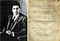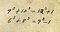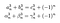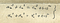# The Hardy-Ramanujan number 1729

“I remember once going to see him when he was ill at Putney. I had ridden in taxi cab number 1729 and remarked that the number seemed to me rather a dull one, and that I hoped it was not an unfavorable omen. “No,” Ramanujan replied, “it is a very interesting number; it is the smallest number expressible as the sum of two cubes in two different ways.” — G.H. Hardy (1918)Left: One of few photographs of Ramanujan. Right: Ramanujan’s manuscript. The representations of 1729 as the sum of two cubes appear in the bottom right corner. Photo: Trinity College library.

The two different ways 1729 is expressible as the sum of two cubes are 1³ + 12³ and 9³ + 10³. The number has since become known as the Hardy-Ramanujan number, the second so-called “taxicab number”, defined as

`Taxicab numbersThe smallest number that can be expressed as the sum of two cubes in n distinct ways.`

So far, six taxicab numbers are known. They are:

`Ta(1) = 2 = 1³ + 1³Ta(2) = 1,729 = 1³ + 12³ = 9³ + 10³Ta(3) = 87,539,319 = 167³ + 436³ = 228³ + 423³ = 255³ + 414³Ta(4) = 6,963,472,309,248 = 2421³ + 19083³ = 5436³ + 18948³ = 10200³ + 18072³ = 13322³ + 16630³Ta(5) = 48,988,659,276,962,496= 38787³ + 365757³ = 107839³ + 362753³ = 205292³ + 342952³ = 221424³ + 336588³ =231518³ + 331954³Ta(6) = 24,153,319,581,254,312,065,344= 582162³ + 28906206³ = 3064173³ + 28894803³ = 8519281³ + 28657487³ = 16218068³ + 27093208³ = 17492496³ + 26590452³ = 18289922³ + 26224366³`

Numbers expressible as the sum of cubes was first mentioned in 1657 by Bernard Frénicle de Bessy, who described the property citing the example of 1729 in his correspondences with John Wallis and Pierre de Fermat. In 1938, Hardy and E. M. Wright proved that such numbers exist for all positive integers n. The next taxicab number, Ta(3) was obtained by computer in 1957 by John Leech. Subsequent numbers were found by Rosendstiel et al (1989), J.A. Dardis (1994) and Hollerbach (2008). For the numbers Ta(7) to T(12) only upper bounds are known, found by Boyer in 2006.

# Ramanujan’s work

Famously, Ramanujan did his work in notebooks which have later been studied extensively with awe by mathematicians and historians alike. As of recently, apart from the mention of the number 1729 in the anecdote above, no further information was known about Ramanujan’s knowledge of the number.

## Diophantine equations

That Ramanujan had done work involving the number 1729 was discovered in one of his manuscripts uncovered in the Library of Trinity College, Cambridge by mathematician Ken Ono and one of his graduate students, Sarah Trebat-Leder. From the single page which is presented completely without notes, it is clear that Ramanujan was working on near-integer solutions to the diophantine equation:

when he presumably stumbled over the case of x = 9, y = 10. Near-integers are numbers that are very close to being an integer, such as e.g. sin(11) = -0.99999206…. Of their discovery in Ramanujan’s manuscripts, Ken Ono later stated:

We were sitting right next to the librarian’s desk, flipping page by page through the Ramanujan box,” recalls Ono. “We came across this one page which had on it the two representations of 1729 [as the sum of cubes]. We started laughing immediately.”Top line: The number 1729 represented by the sum of two cubes, in two ways

What the two spotted was not the number 1729 itself, but rather the number in its two cube sum representations 9³+10³ = ¹³ + 1²³, which Ramanujan had come across in his investigations of near-integer solutions to equation 1 above.

## Fermat’s Last Theorem

Ono and Trebat-Leder’s discovery was amusing because equation 1 above, of course, is the well-known equation (for n = 3) from Fermat’s Last Theorem, the famous conjecture proposed by French mathematician Pierre de Fermat in 1637:

`Fermat's Last TheoremThere are no three positive integers a, b, c that satisfy the equation aⁿ + bⁿ = cⁿ for any integer value of n greater than 2.`

Although the particular case studied by Ramanujan (n = 3) was shown to have no solutions by Leonard Euler in 1770 (albeit with a large gap), the general case of n > 2 was still very much unsolved at the time of Ramanujan, not amenable to solution until Andrew Wiles famously proved the conjecture in 1994. The relationship between taxicab numbers and Fermat’s Last Theorem may be described in the following manner:

`For aⁿ + bⁿ = cⁿ, let n = 3. Then Fermat's Last Theorem states that there cannot exist a taxicab number Ta(x) such that the number c = ³√Ta(x) is an integer.`

## Euler’s Diophantine equation

The diophantine equation (describing near-integer solutions to Fermat’s Last theorem) which Ramanujan was working on was previously studied by Euler, and is sometimes called the Euler diophantine equation:

On the same page still, above Ramanujan’s examples of solutions to the equation, he also presented three functions, along with expansions in powers of x (about the origin) and in powers of ξ (about infinity).

From the manuscript, the three functions and their expansions in powers of x are:

For which the coefficients of a, b, c for the first few values of n are:

`n = 0: a₀ = 1, b₀ = 2, c₀ = 2n = 1: a₁ = 135, b₁ = 138, c₁ = 172n = 2: a₂ = 11161, b₂ = 11468, c₂ = 14258n = 3: a₃ = 926271, b₃ = 951690, c₃ = 1183258n = 4: a₄ = 76869289, b₄ = 78978818, c₄ = 98196140n = 5: a₅ = 6379224759, b₅ = 6554290188, c₅ = 8149096378`

The three functions and their expansions in powers of ξ are:

For which the coefficients of α, β, γ for the first few values of n are:

`n = 0: α₀ = 9, β₀ = -12, γ₀ = -10n = 1: α₁ = 791, β₁ = -1010, γ₁ = -812n = 2: α₂ = 65601, β₂ = -83802, γ₂ = -67402n = 3: α₃ = 5444135, β₃ = -6954572, γ₃ = -5593538n = 4: α₄ = 451797561, β₄ = -577145658, γ₄ = -464196268n = 5: α₅ = 37493753471, β₅ = -47896135058, γ₅ = -38522696690`

In addition, Ramanujan provided two general expressions, adapted from Euler’s Diophantine equation, for generating near-integer solutions to Fermat’s Last theorem for n = 3:Equations 5 and 6. Ramanujan’s equations for near-integer solutions to the diophantine equation a³ + b³ = c³

What Ono and Trebat-Leder had discovered, in other words, was that the Hardy-Ramanujan number, 1729 was known to Ramanujan as a solution to equation 6 above, expressible as the expansion of powers of ξ, given by the coefficients α, β, γ for n = 0, namely α₀ = 9, β₀ = −12, γ₀ = −10.Ramanujan’s equations for providing near-integer solutions to the Euler diophantine equation

And so, when Hardy came to see Ramanujan in the hospital at Putney that day in 1918, not only did Ramanujan recognize the number’s properties, he also knew the formula for generating infinitely many numbers with the same properties, given by equation 5 and 6 above. Amazing.

“Every positive integer was one of Ramanujan’s personal friends.” — G. E. Littlewood

This essay is part of a series of stories on math-related topics, published in Cantor’s Paradise, a weekly Medium publication. Thank you for reading!

Written by

Written by

## Jørgen Veisdal

#### Editor-in-Chief at Cantor’s Paradise. Research Fellow at the Norwegian University of Science and Technology.